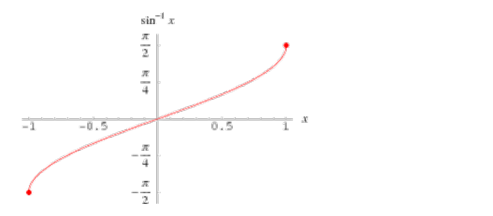# Mark the tick against the correct answer in the following:

Question:

Mark the tick against the correct answer in the following:

Range of $\sin ^{-1} x$ is

A. $\left[0, \frac{\pi}{2}\right]$

B. $[0, \pi]$

C. $\left[\frac{-\pi}{2}, \frac{\pi}{2}\right]$

D. None of these

Solution:

To Find: The range of $\sin ^{-1} x$

Here,the inverse function is given by $y=f^{-1}(x)$

The graph of the function $y=\sin ^{-1}(x)$ can be obtained from the graph of

$Y=\sin x$ by interchanging $x$ and $y$ axes.i.e, if $(a, b)$ is a point on $Y=\sin x$ then $(b, a)$ is

The point on the function $y=\sin ^{-1}(x)$

Below is the Graph of range of $\sin ^{-1}(x)$From the graph, it is clear that the range of $\sin ^{-1}(x)$ is restricted to the interval

$\left[-\frac{\pi}{2}, \frac{\pi}{2}\right]$